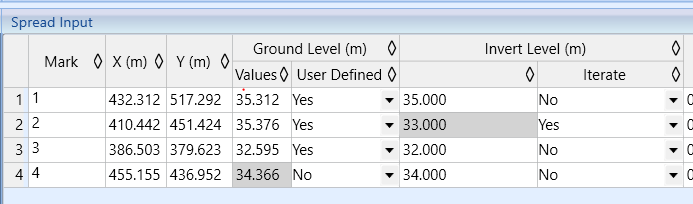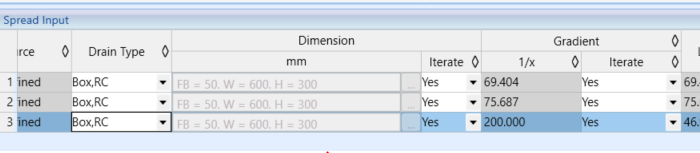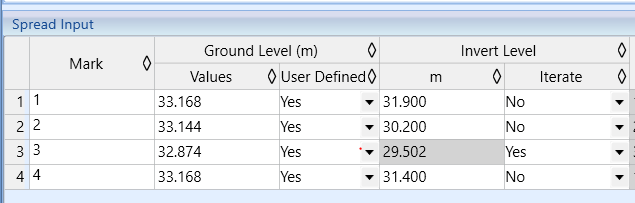603 - 5885 1250 (4 lines) info@mes100.com
Select Page

Users might have encountered a situation whereby at one node, one needs to manually set the different invert levels at different pipe ends . Such a situation typically arises when engineers need to readjust back the pipe invert level/gradient to tally with on-site condition.

MiTS currently provides users to insert the invert level per pipe basis (upstream and downstream of the pipe, instead of at one node), which then calculate the gradient and drop depth for you. The following examples illustrate and compare the new and old versions.

DRAINAGE

The example below is taken from this example project file (new version) and project file 2 (old version). There are multiple inflows and the invert level of each of the sump has drops.

Previous versionAbove is how the previous versions work, whereby users have to first calculate the invert level manually, in case where drops is required. Since the invert level is user-defined, thus the gradient also has to be manually calculated in order to adjust the following sump invert level.Image above is the drainage taken from the previous version project. As you notice, users now can straight away insert the invert level of the upstream and downstream of the pipe, without the hurdle of calculating the drop and gradient manually.You can set the gradient iteration to “Yes” as software will calculate based on the invert level assigned at the upstream and downstream of a pipe.

We also provide a benchmark to compare between manual calculation with the calculation of the software.

SEWERAGE

Sewerage module also works the same as drainage, where users can determine the invert level based on pipe basis. Users no longer need to calculate the drop, gradient and invert level manually.

Previous versionPrevious version requires users to insert the invert level and calculate the gradient manually if certain invert level of sump at a pipe is required.